2018-04-23 10:54:22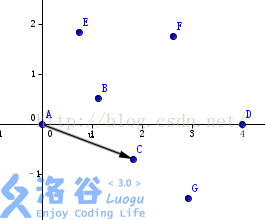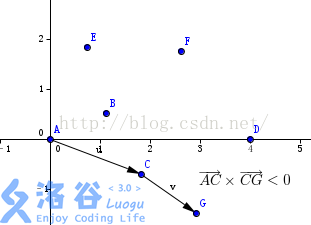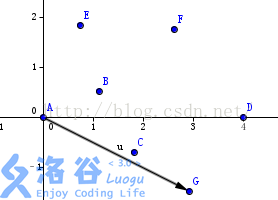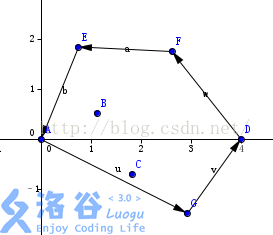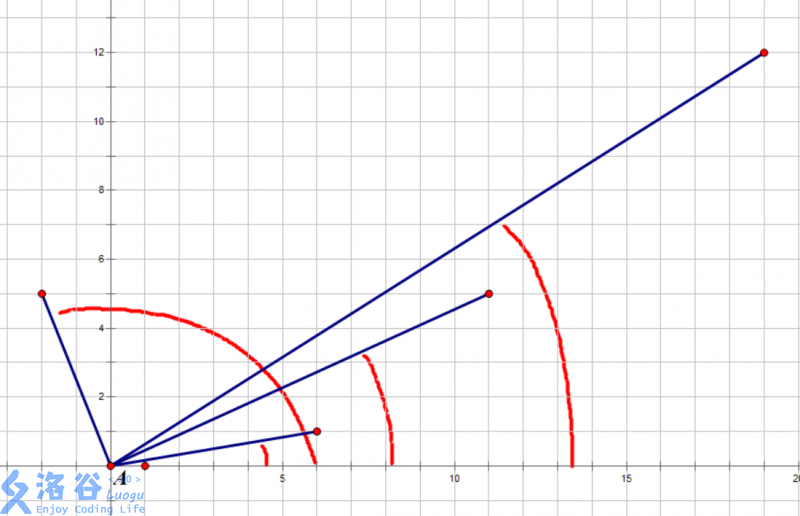1.把所有点放在二维坐标系中，则纵坐标最小的点一定是凸包上的点

2.把所有点的坐标平移一下，使 A 作为原点

3.计算各个点相对于 A 的幅角 α ，按从小到大的顺序对各个点排序。当 α 相同时，距离 A 比较近的排在前面。我们由几何知识可以知道，结果中第一个点 B 和最后一个点一定是凸包上的点。

（以上是准备步骤，以下开始求凸包）

4.连接s[top-1]和s[top]这两个点，得到直线 L 。看当前点是在直线 L 的右边还是左边。如果在直线的右边就执行步骤5；如果在直线上，或者在直线的左边就执行步骤6。

5.如果在右边，则s[top]不是凸包上的点，把栈顶元素出栈。执行步骤4。

6.当前点是凸包上的点，把它压入栈，执行步骤7。

7.检查当前点是不是步骤3那个结果的最后一个元素。是最后一个元素的话就结束。如果不是的话就把 P2 后面那个点做当前点，返回步骤4。

#include<cstdio>
#include<cstring>
#include<iostream>
#include<algorithm>
using namespace std;
int n;
struct node
{
int x,y;
}p,s;
int cross(node a,node b,node c)//计算叉积
{
return (b.x-a.x)*(c.y-a.y)-(c.x-a.x)*(b.y-a.y);
}
int dis(node a,node b)
{
return (a.x-b.x)*(a.x-b.x)+(a.y-b.y)*(a.y-b.y);
}
bool cmp1(node a,node b)
{
if(a.y==b.y) return a.x<b.x;
return a.y<b.y;
}
bool cmp2(node a,node b)//极角排序
{
int k=cross(s,a,b);
if(k>0) return 1;
else if(k==0 && dis(s,a)<=dis(s,b)) return 1;//共线的话就把距离近的排在前面
else return 0;
}
int main()
{
scanf("%d",&n);
for(int i=1;i<=n;i++)
scanf("%d%d",&p[i].x,&p[i].y);
sort(p+1,p+n+1,cmp1);//按横纵坐标排序，得出最左下角的点
s=p;
sort(p+2,p+n+1,cmp2);//极角排序
s=p;//得到辐角最小的点，入栈
int top=2;
for(int i=3;i<=n;i++)
{
while(cross(s[top-1],s[top],p[i])<0) top--;//比较当前点在直线的右边还是左边。在右边就一直弹栈顶
s[++top]=p[i];//进栈
}
for(int i=1;i<=top;i++)
cout<<s[i].x<<" "<<s[i].y<<endl;
return 0;
}
• star
首页